# Python3网络爬虫——（4）正则表达式（re模块）

1、re.match函数

2、re.search函数

3、re.findall函数

4、re.sub函数

5、re.compile函数

6、re.finditer函数

7、re.split函数

## 一、正则表达式

1、正则表达式是字符串搜索引擎

Python正则表达式是对字符串操作的一种逻辑公式，就是用事先定义好的一些特定字符、及这些字符的组合，组成一个“规则字符串”，这个“规则字符串”用来表达对字符串的一种过滤逻辑。

2、正则表达式在线测试工具

3、常见匹配模式：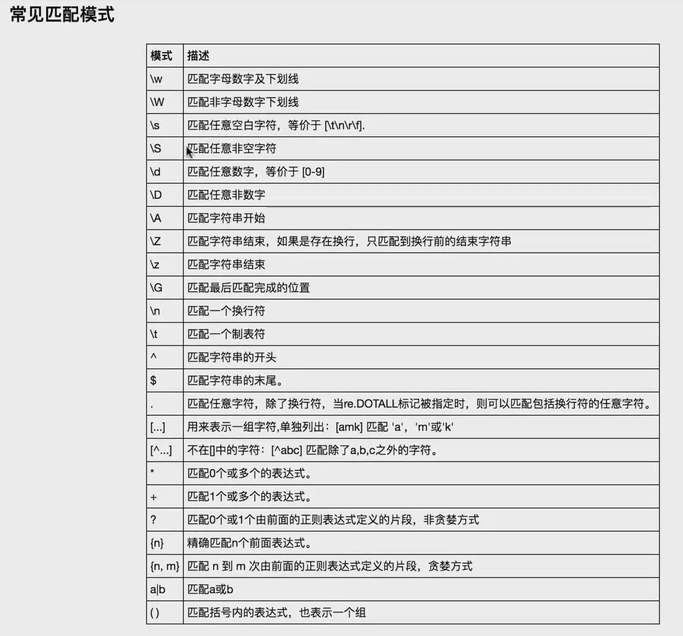4、正则表达式修饰符 - 可选标志

re.I使匹配对大小写不敏感
re.L做本地化识别（locale-aware）匹配
re.M多行匹配，影响 ^ 和 $re.S使 . 匹配包括换行在内的所有字符 re.U根据Unicode字符集解析字符。这个标志影响 \w, \W, \b, \B. re.X该标志通过给予你更灵活的格式以便你将正则表达式写得更易于理解。 ## 二、Python语言中的re模块拥有全部的正则表达式功能 ### 1、re.match函数 • re.match 尝试从字符串的起始位置匹配一个模式，如果不是起始位置匹配成功的话，match()就返回none。 • 函数语法re.match(pattern, string, flags=0) • 函数参数说明：  参数 描述 pattern 匹配的正则表达式 string 要匹配的字符串。 flags 标志位，用于控制正则表达式的匹配方式 • 匹配成功re.match方法返回一个匹配的对象，否则返回None。 • 我们可以使用group(num) 或 groups() 匹配对象函数来获取匹配表达式。  匹配对象方法 描述 group(num=0) 匹配的整个表达式的字符串，group() 可以一次输入多个组号，在这种情况下它将返回一个包含那些组所对应值的元组。 groups() 返回一个包含所有小组字符串的元组，从 1 到 所含的小组号。 （1）最常规的匹配 import re content='Hello 123 4567 World_This is a Regex Demo' result=re.match('^Hello\s\d\d\d\s\d{4}\s\w{10}.*Demo$',content)
print(len(content))
print(result)
print(result.group()) #输出匹配的整个表达式字符串
print(result.span()) #输出匹配结果的范围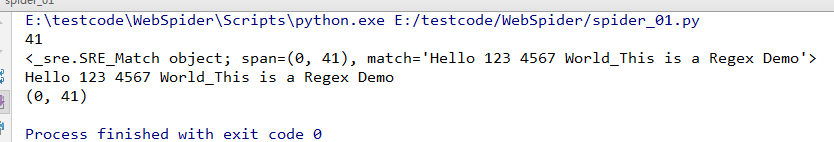（2）泛匹配

import re
content='Hello 123 4567 World_This is a Regex Demo'
result=re.match('^Hello.*Demo$',content) print(result.group()) #输出匹配的整个表达式字符串 结果为：Hello 123 4567 World_This is a Regex Demo （3）匹配目标 import re content='Hello 1234567 World_This is a Regex Demo' result=re.match('^Hello\s(\d+)\sWorld.*Demo$',content)
print(result.group()) #输出匹配的整个表达式字符串
print(result.group(1))#获取匹配目标（即小括号内的字符串）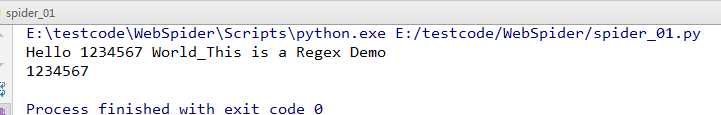（4）贪婪匹配

import re
content='Hello 1234567 World_This is a Regex Demo'
result=re.match('^He.*(\d+).*Demo$',content)# .*匹配尽可能多的字符 print(result.group()) #输出匹配的整个表达式字符串 print(result.group(1))#获取匹配目标（即小括号内的字符串）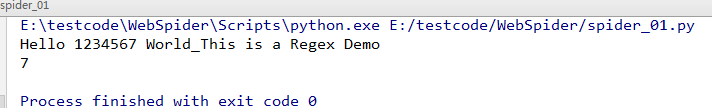（5）非贪婪匹配 import re content='Hello 1234567 World_This is a Regex Demo' result=re.match('^He.*?(\d+).*Demo$',content)#  .*？匹配尽可能少的字符
print(result.group()) #输出匹配的整个表达式字符串
print(result.group(1))#获取匹配目标（即小括号内的字符串）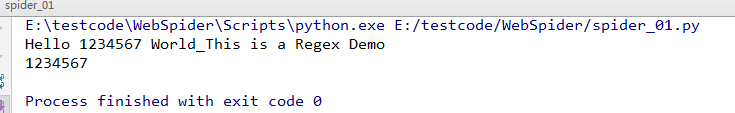（6）匹配模式

import re
content='Hello 1234567 World_This ' \
'is a Regex Demo'
result=re.match('^He.*?(\d+).*?Demo$',content,re.S) #re.S匹配换行符 print(result.group()) #输出匹配的整个表达式字符串 print(result.group(1))#获取匹配目标（即小括号内的字符串）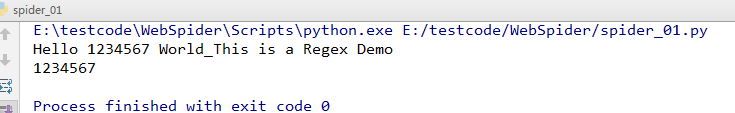（7）转义 import re content='price is$5.00'
result=re.match('price is \$5\.00',content) #特殊字符用转义 print(result.group()) #price is$5.00

### 2、re.search函数

• re.search 扫描整个字符串并返回第一个成功的匹配。
• 函数语法：re.search(pattern, string, flags=0)
• 函数参数说明：
 参数 描述 pattern 匹配的正则表达式 string 要匹配的字符串。 flags 标志位，用于控制正则表达式的匹配方式，如：是否区分大小写，多行匹配等等。
• 匹配成功re.search方法返回一个匹配的对象，否则返回None。
• 我们可以使用group(num) 或 groups() 匹配对象函数来获取匹配表达式。
 匹配对象方法 描述 group(num=0) 匹配的整个表达式的字符串，group() 可以一次输入多个组号，在这种情况下它将返回一个包含那些组所对应值的元组。 groups() 返回一个包含所有小组字符串的元组，从 1 到 所含的小组号。

import re
content='Extra strings Hello 1234567 World_This is a Regex Demo Extra strings'
result=re.search('Hello.*?(\d+).*?Demo',content)
print(result)
print(result.group(1))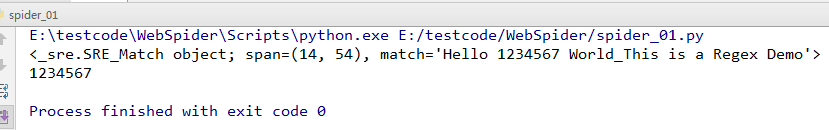re.match只匹配字符串的开始，如果字符串开始不符合正则表达式，则匹配失败，函数返回None；而re.search匹配整个字符串，直到找到一个匹配

### 3、re.findall函数

• re.findall搜索字符串，在字符串中找到正则表达式所匹配的所有子串，并返回一个列表，如果没有找到匹配的，则返回空列表。

• 语法格式为：findall(string[, pos[, endpos]])

• string : 待匹配的字符串。
• pos : 可选参数，指定字符串的起始位置，默认为 0。
• endpos : 可选参数，指定字符串的结束位置，默认为字符串的长度。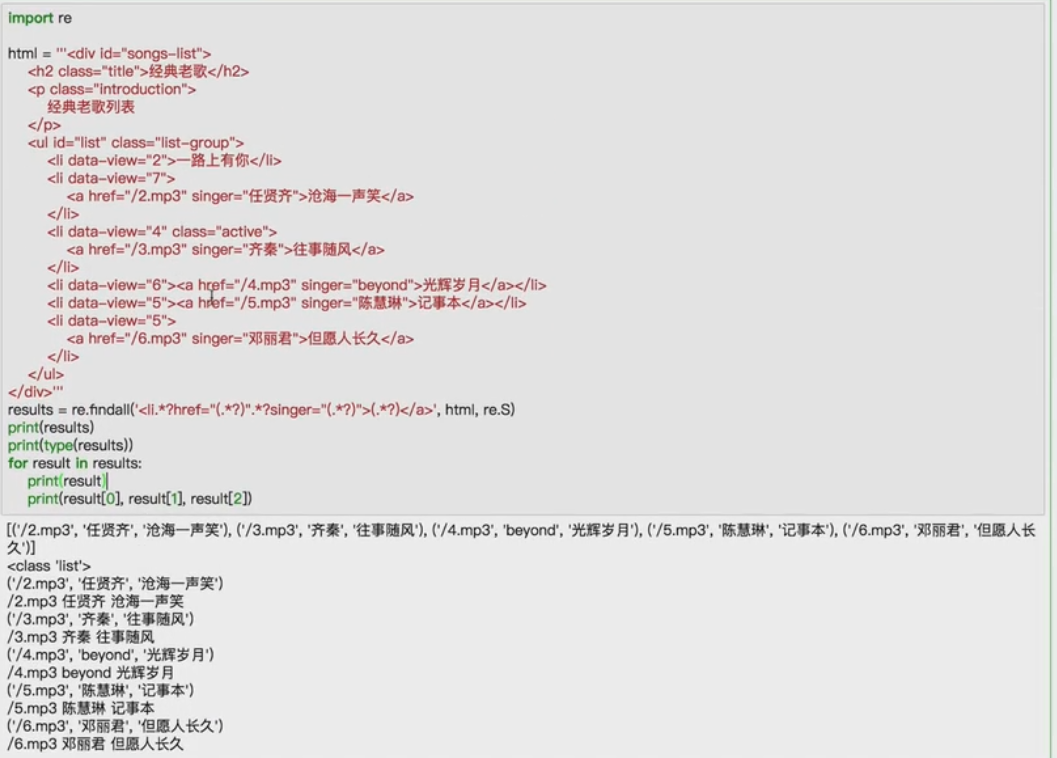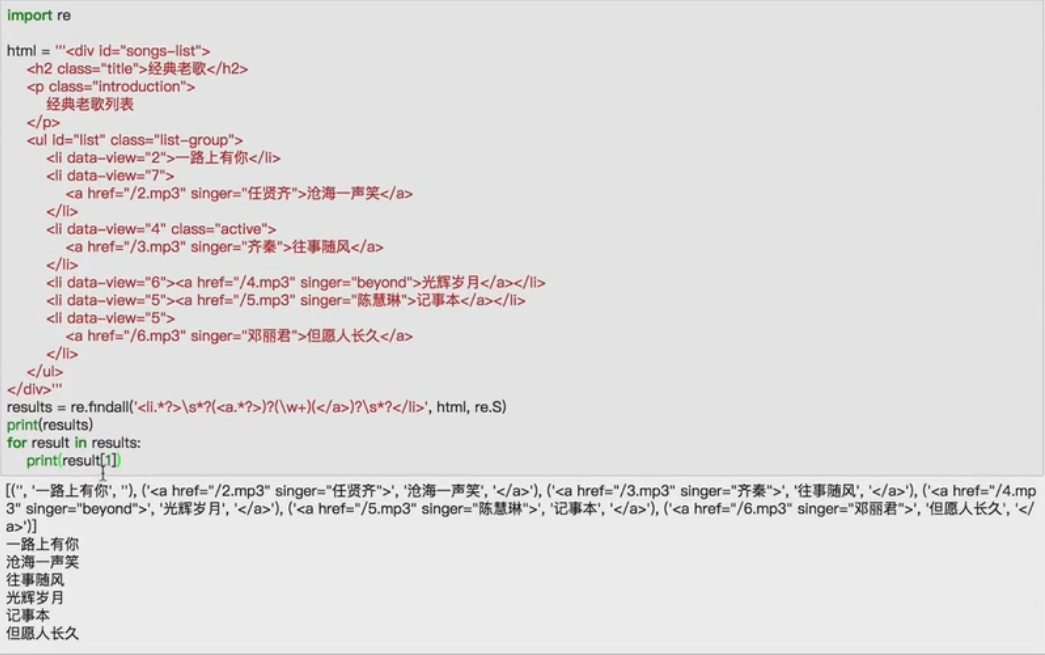### 4、re.sub函数

• re.sub 替换字符串中每一个匹配的子串后返回替换后的字符串。
• 语法：re.sub(pattern, repl, string, count=0, flags=0)

• pattern : 正则中的模式字符串。
• repl : 替换的字符串，也可为一个函数。
• string : 要被查找替换的原始字符串。
• count : 模式匹配后替换的最大次数，默认 0 表示替换所有的匹配。
import re
content='Extra strings Hello 1234567 World_This is a Regex Demo Extra strings'
content1=re.sub('\d+','',content)
print(content1)
content2=re.sub('\d+','Replacement',content)
print(content2)                                              #r 表示字符串为非转义的原始字符串
content3=re.sub('(\d+)',r'\1 8910',content)  # \1是把第一个括号里的内容拿过来
print(content3)### 5、re.compile函数

• compile 函数用于编译正则表达式，生成一个正则表达式（ Pattern ）对象，供 match() 和 search() 这两个函数使用。
• 语法格式为：re.compile(pattern[, flags])

• pattern : 一个字符串形式的正则表达式
import re
content='Hello 1234567 World_This ' \
'is a Regex Demo'
pattern=re.compile('Hello.*Demo',re.S)
result=re.match(pattern,content)
print(result)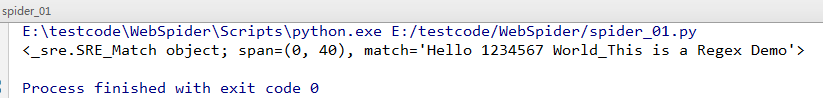### 6、re.finditer函数

re.finditer(pattern, string, flags=0)

 参数 描述 pattern 匹配的正则表达式 string 要匹配的字符串。 flags 标志位，用于控制正则表达式的匹配方式，如：是否区分大小写，多行匹配等等
import re
content='12a32bc43jf3'
it=re.finditer(r'\d+',content)
for mat in it:
print(mat.group())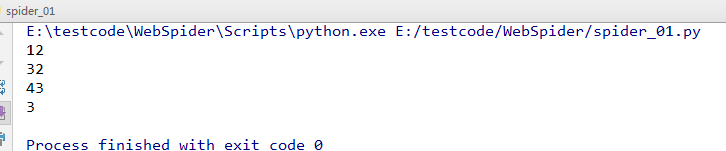### 7、re.split函数

re.split(pattern, string[, maxsplit])按照能够匹配的子串将string分割后返回列表。maxsplit用于指定最大分割次数，不指定将全部分割。
import re
content='one1two2three3four4'
result=re.split(r'\d+',content)
print(result)    #['one', 'two', 'three', 'four', '']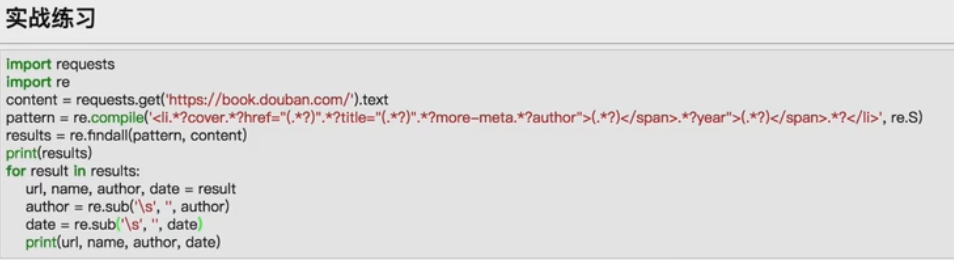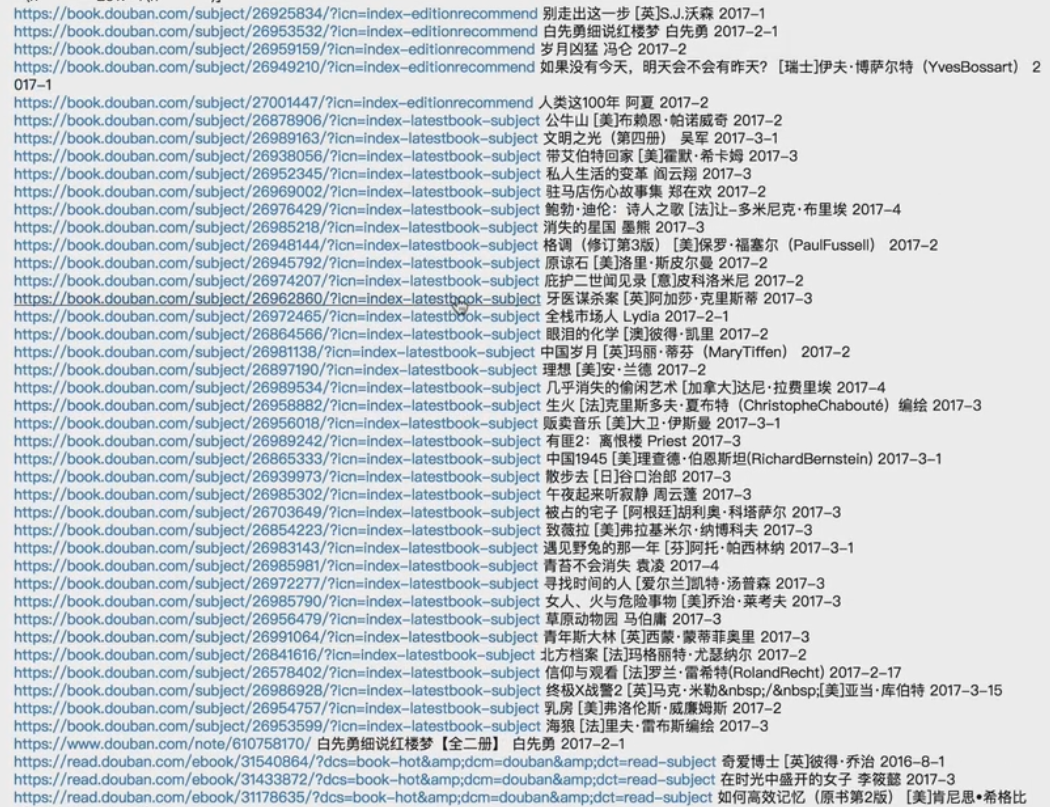## 三、常用正则表达式

1、时间正则

• pattern1 = re.compile('\d{1,2}[:|：]\d{1,2}')
• pattern2 = re.compile('(周[一-日|1-7])')
• pattern3 = re.compile('(早晨|上午|中午|下午|晚上)')
• pattern4 = re.compile('(第[1-9|一-九]节课)')
• pattern5 = re.compile('([明|今|后][天|日])')
• pattern6 = re.compile('([一-十二|\d][点|时])')

2、只保留中英文和数字的正则

• pattern1 = re.compile('[^\u4e00-\u9fa5^a-z^A-Z^0-9]')

维基百科正则表达式规则总结07-243万+03-31112
04-126941
07-197006
04-182642
05-255万+
06-25604
04-28320
07-23441
05-305828
05-20
09-18
01-171万+
08-2310万+
09-28